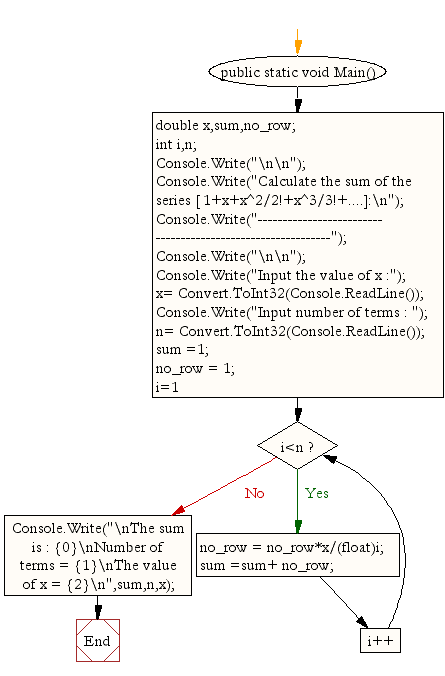﻿ C# - Calculate the sum of the series [ 1+x+x^2/2!+x^3/3!+..]# C# Sharp Exercises: Calculate the sum of the series [ 1+x+x^2/2!+x^3/3!+....]

## C# Sharp For Loop: Exercise-23 with Solution

Write a program in C# Sharp to display the sum of the series [ 1+x+x^2/2!+x^3/3!+....].

Sample Solution:-

C# Sharp Code:

``````using System;
public class Exercise23
{
public static void Main()
{
double x,sum,no_row;
int i,n;

Console.Write("\n\n");
Console.Write("Calculate the sum of the series [ 1+x+x^2/2!+x^3/3!+....]:\n");
Console.Write("------------------------------------------------------------");
Console.Write("\n\n");

Console.Write("Input the value of x :");
Console.Write("Input number of terms : ");
sum =1; no_row = 1;
for (i=1;i<n;i++)
{
no_row = no_row*x/(float)i;
sum =sum+ no_row;
}
Console.Write("\nThe sum  is : {0}\nNumber of terms = {1}\nThe value of x = {2}\n",sum,n,x);
}
}
```
```

Sample Output:

```Calculate the sum of the series [ 1+x+x^2/2!+x^3/3!+....]:
------------------------------------------------------------
Input the value of x :5
Input number of terms : 5
The sum  is : 65.375
Number of terms = 5
The value of x = 5
```

Flowchart:C# Sharp Code Editor:

Contribute your code and comments through Disqus.

What is the difficulty level of this exercise?

Test your Programming skills with w3resource's quiz.

﻿